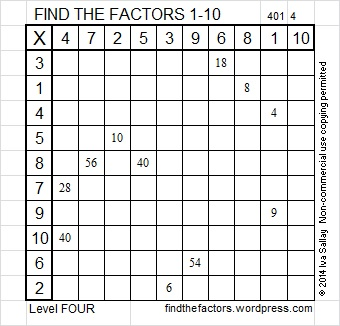# 26 and Level 4

26 is a composite number. 26 = 1 x 26 or 2 x 13. Factors of 26: 1, 2, 13, 26. Prime factorization: 26 = 2 x 13.26 is never a clue in the FIND THE FACTORS 1-10 or 1-12 puzzles.Write the numbers 1-12 in the top row and again in the first column so that those numbers are the factors of the given clues. Use logic, not guessing and checking, to find its unique solution. Here is how to solve this level 4 “snow shovel” puzzle:

Begin by looking for perfect square clues, 1, 25, 49, 64, 81, 100, 121, or 144 because there is only one way to factor any of them so that both factors are numbers from 1 to 12. If you find one of those perfect square clues, write down its factors in the corresponding factor row and factor column.

Next locate a row or a column with at least 2 clues. Find the common factor of that row or column that will allow you to write only numbers from 1 to 12 in both the factor row and the factor column. If there is more than one row or column with at least 2 clues, repeat the previous step until only rows and columns with one clue remain.

Now you have at least 3 factors written down. Next look at ALL the remaining clues in the puzzle and find one that can only be factored using one of the factors you’ve written down already but haven’t used twice. Repeat the last step until all (or almost all) of the factors are found.

If a row or a column contains no clues, use logic is determine which number from 1 to 12 should be written there.

While working on these puzzles, occasionally count from 1 to 12 to make sure each of those numbers is written exactly once in both the factor row and the factor column. You will notice a rhythm for the answers as you work. Good luck!

This week’s puzzles are also available in an excel file here, if you have a spreadsheet program on your computer. If you enable editing in excel, you can type your answers directly onto the puzzle, and you can also easily print the puzzles.

Here is the solution to the week 1 level 4 puzzle: